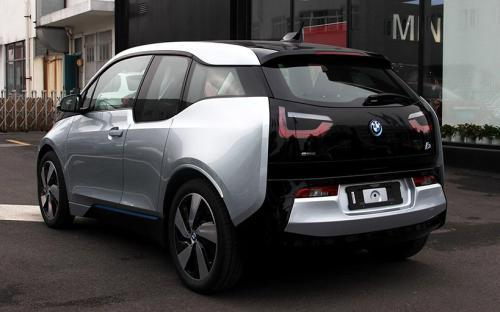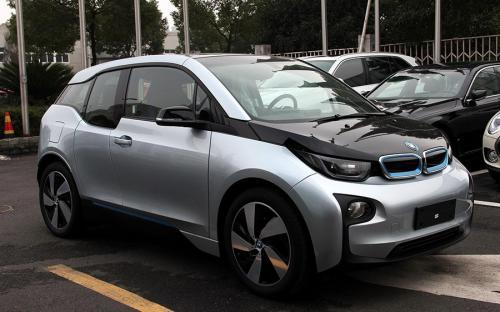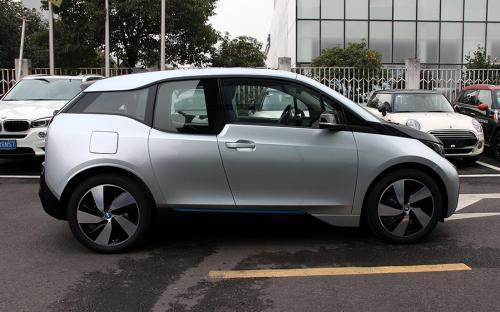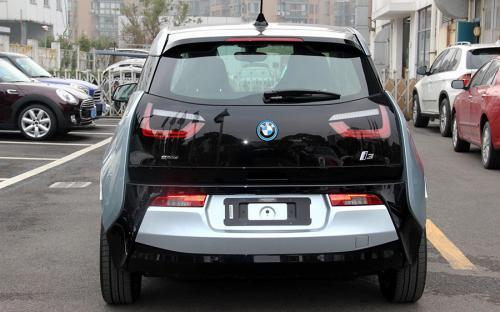### 宝马(进口) 宝马i32016款最低售价：42.28 万元起

4006(mm)1775(mm)1600(mm)##### 配置亮点：
• 胎压监测装置

• ISOFIX儿童座椅接口

• 车身稳定控制(ESC/ESP/DSC等)

• 电动天窗

• 定速巡航

• 后倒车雷达

• 真皮座椅

• GPS导航系统

• 氙气大灯

• 后视镜加热

• 提交
2016款 升级款 豪华型 (107张)

宝马(进口) 宝马i3 绕车实拍• 宝马(进口) 宝马i3 在售车型

排量 车型 厂商指导价 本地最低报价 购车工具
k
升级款 时尚型 纯电动 电动车单速变速箱
42.28万
升级款 豪华型 增程式 电动车单速变速箱
45.58万
升级款 增程型 纯电动 电动车单速变速箱
52.28万

宝马(进口) 宝马i3 经销商

查看更多 >>

### 宝马(进口) 宝马i3 动力加速

宝马i3 0-100公里加速时间分布在 7.3-8.1秒 属于 运动级

动力级别 加速时间 车型
运动级(3款)
7.3s
升级款 时尚型
8.1s
升级款 豪华型
7.9s
升级款 增程型

宝马(进口) 宝马i3 视频

宝马(进口) 宝马i3 新闻资讯

# 充电更快 宝马推i Wallbox Pro充电桩

新闻 超过4154次关注

近日，宝马正式推出了一款具有诸多智能功能的“iWallboxPro”充电桩，其将提供给宝马i系列车型进行充电。据悉，这个充电桩将比此前的“iWallboxPure”充电桩充电速度更快。

# 运动气息浓厚 宝马i3外观改装案例介绍

改装 超过3735次关注

日本宝马改装专家3DDesign和泰国改装公司StudieThailand近日联手打造了一辆宝马i3，为这款车设计了非常醒目的涂装和外观包围套件。

# 北美车展亮相 宝马新款i3配置将升级

新闻 超过4100次关注

近日，宝马美国公司生产部门的相关人员向媒体透露，宝马将会在明年推出新款i3车型，新车的配置将会有所升级，其将会在明年1月份的北美车展上正式亮相。

# 将于9月进口国内 宝马i3/i8新能源车型

新闻 超过5805次关注

近日，我们得到消息，宝马i3和i8车型将于今年9月在国内正式上市。宝马官方已经表示，将在国内建设包括充电服务设施、充电方案以及出行等服务项目以确保新能源车型在...

# 品牌国内个人充电服务 宝马推进BMW i

新闻 超过5460次关注

日前，宝马中国公布了BMWi标准化的服务流程，据悉，华商三优及其子公司成为首批BMWi电动汽车充电设备安装服务供应商。

# 宝马将扩产碳纤维部件满足更多车型

技术 超过5720次关注

日前，我们从宝马官方获悉，宝马将在2014年-2015年对其德国Landshut工厂投入四亿欧元用于扩产，该工厂主要生产铝镁合金和碳纤维增强复合材料（CFRP）部件。

# 宝马i3/i8将9月21日上市 45万/200万起

新闻 超过7004次关注

日前，我们获得消息宝马i3和i8将会于9月21日正式上市，其中i3的预计起售价为45万，i8的预计起售价为200万元左右。目前宝马已经在北京、上海、深圳和沈阳正式授权7家...

# 宝马i3自动泊车系统体验 可无人驾驶？

导购 超过7632次关注

还在担心身边女性车主的停车难问题么？你的车有自动泊车？那又怎样，竟然还需要“瞄准”？手动切换挡位？没有自动刹停？老实说，我一直认为自动泊车是个可有可无的...

# 奔驰将推全新纯电动车型 与宝马i3竞争

导购 超过4950次关注

近日，有海外媒体报道，奔驰计划推出一款全新的纯电动车型，新车将以宝马i3为主要竞争对手。

猜你喜欢

﻿
• 快速找车
• 选择品牌
• 选择品牌
• A  奥迪
• A  阿斯顿·马丁
• A  阿尔法·罗密欧
• B  宝沃
• B  布加迪
• B  巴博斯
• B  保时捷
• B  宾利
• B  奔驰
• B  宝马
• B  本田
• B  别克
• B  标致
• B  比亚迪
• B  宝骏
• B  北汽制造
• B  北汽新能源
• B  北汽幻速
• B  北汽威旺
• B  北京汽车
• B  奔腾
• B  北汽绅宝
• C  长安
• C  长安商用
• C  长城
• C  昌河
• D  大众
• D  道奇
• D  DS
• D  东南
• D  东风风神
• D  东风风行
• D  东风小康
• D  东风风度
• D  东风
• F  福特
• F  丰田
• F  菲亚特
• F  法拉利
• F  福田
• F  福迪
• F  福汽启腾
• G  观致
• G  广汽传祺
• G  广汽吉奥
• G  GMC
• H  红旗
• H  汉腾汽车
• H  哈弗
• H  哈飞
• H  海格
• H  海马
• H  华颂
• H  黄海
• H  华泰
• H  恒天
• J  吉利汽车
• J  捷豹
• J  Jeep
• J  江淮
• J  江铃
• J  金杯
• J  九龙
• J  金旅
• K  凯翼
• K  凯迪拉克
• K  克莱斯勒
• K  科尼塞克
• K  卡威
• K  开瑞
• L  路虎
• L  林肯
• L  劳斯莱斯
• L  兰博基尼
• L  雷克萨斯
• L  铃木
• L  雷诺
• L  理念
• L  力帆
• L  莲花汽车
• L  猎豹
• L  路特斯
• L  陆风
• M  马自达
• M  MG
• M  MINI
• M  玛莎拉蒂
• M  摩根
• M  迈凯轮
• N  纳智捷
• O  欧宝
• O  讴歌
• O  欧朗
• Q  奇瑞
• Q  起亚
• Q  启辰
• R  日产
• R  荣威
• R  瑞麒
• S  三菱
• S  斯威汽车
• S  萨博
• S  smart
• S  斯柯达
• S  斯巴鲁
• S  思铭
• S  双龙
• S  上汽大通
• S  双环
• T  特斯拉
• T  腾势
• W  沃尔沃
• W  五菱汽车
• W  五十铃
• W  威兹曼
• W  威麟
• X  现代
• X  雪佛兰
• X  雪铁龙
• X  西雅特
• Y  一汽
• Y  英菲尼迪
• Y  英致
• Y  依维柯
• Y  野马汽车
• Y  永源
• Z  众泰
• Z  中华
• Z  中兴
• Z  知豆
• 选择车系
• 选择车系
• 车型对比
• 选择品牌
• 选择品牌
• A  奥迪
• A  阿斯顿·马丁
• A  阿尔法·罗密欧
• B  宝沃
• B  布加迪
• B  巴博斯
• B  保时捷
• B  宾利
• B  奔驰
• B  宝马
• B  本田
• B  别克
• B  标致
• B  比亚迪
• B  宝骏
• B  北汽制造
• B  北汽新能源
• B  北汽幻速
• B  北汽威旺
• B  北京汽车
• B  奔腾
• B  北汽绅宝
• C  长安
• C  长安商用
• C  长城
• C  昌河
• D  大众
• D  道奇
• D  DS
• D  东南
• D  东风风神
• D  东风风行
• D  东风小康
• D  东风风度
• D  东风
• F  福特
• F  丰田
• F  菲亚特
• F  法拉利
• F  福田
• F  福迪
• F  福汽启腾
• G  观致
• G  广汽传祺
• G  广汽吉奥
• G  GMC
• H  红旗
• H  汉腾汽车
• H  哈弗
• H  哈飞
• H  海格
• H  海马
• H  华颂
• H  黄海
• H  华泰
• H  恒天
• J  吉利汽车
• J  捷豹
• J  Jeep
• J  江淮
• J  江铃
• J  金杯
• J  九龙
• J  金旅
• K  凯翼
• K  凯迪拉克
• K  克莱斯勒
• K  科尼塞克
• K  卡威
• K  开瑞
• L  路虎
• L  林肯
• L  劳斯莱斯
• L  兰博基尼
• L  雷克萨斯
• L  铃木
• L  雷诺
• L  理念
• L  力帆
• L  莲花汽车
• L  猎豹
• L  路特斯
• L  陆风
• M  马自达
• M  MG
• M  MINI
• M  玛莎拉蒂
• M  摩根
• M  迈凯轮
• N  纳智捷
• O  欧宝
• O  讴歌
• O  欧朗
• Q  奇瑞
• Q  起亚
• Q  启辰
• R  日产
• R  荣威
• R  瑞麒
• S  三菱
• S  斯威汽车
• S  萨博
• S  smart
• S  斯柯达
• S  斯巴鲁
• S  思铭
• S  双龙
• S  上汽大通
• S  双环
• T  特斯拉
• T  腾势
• W  沃尔沃
• W  五菱汽车
• W  五十铃
• W  威兹曼
• W  威麟
• X  现代
• X  雪佛兰
• X  雪铁龙
• X  西雅特
• Y  一汽
• Y  英菲尼迪
• Y  英致
• Y  依维柯
• Y  野马汽车
• Y  永源
• Z  众泰
• Z  中华
• Z  中兴
• Z  知豆
• 选择车系
• 选择车系
• 选择车型
• 选择车型
• 意见反馈